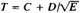# Geiger-Nuttall Law

(redirected from Geiger-Nuttall rule)

## Geiger-Nuttall Law

a law that establishes the connection between the probability of alpha decay of a nucleus and the energy of alpha particles; it is expressed by the formula log, where E is the energy of the alpha particles in mega electron volts, T is the half-life in seconds, and C and D are constants.

The Geiger-Nuttall law was established experimentally in 1911-12 by H. Geiger and J. M. Nuttall and was later explained theoretically (1928). It is the most accurate description of the alpha decay of even-even nuclei of isotopes of the same element. For nuclei with different values of Z (Z is the atomic number of the element), the constants C and D are slightly different—for example, for Z = 84, C = —50.15 and D = 128.8; for Z = 86, C = -50.94 and D = 132.7.

Site: Follow: Share:
Open / Close